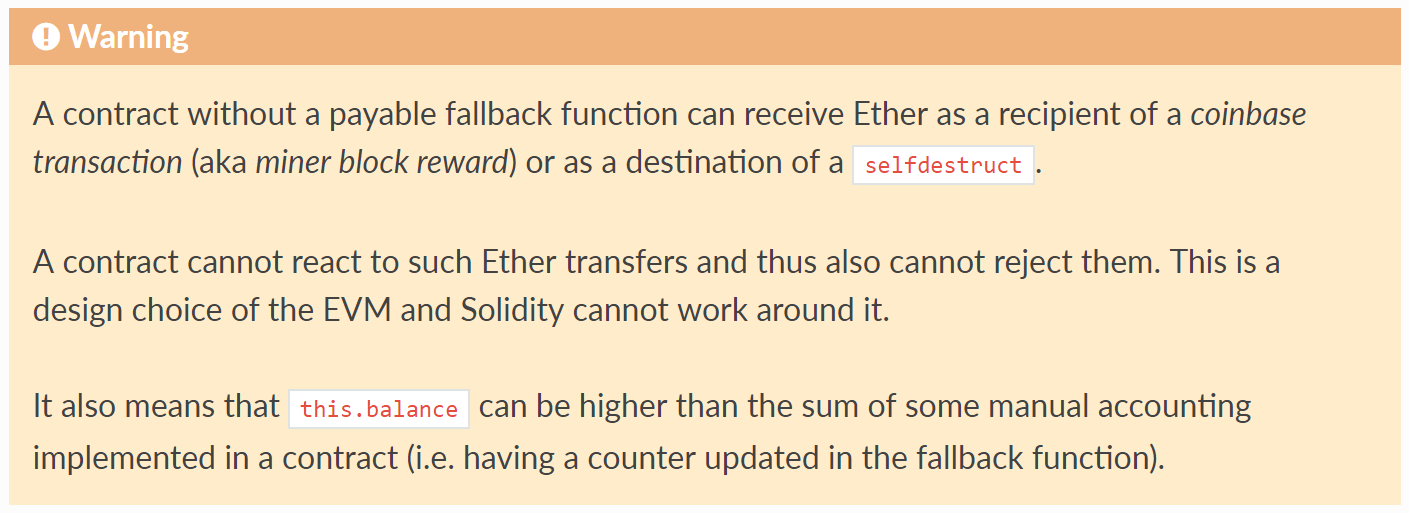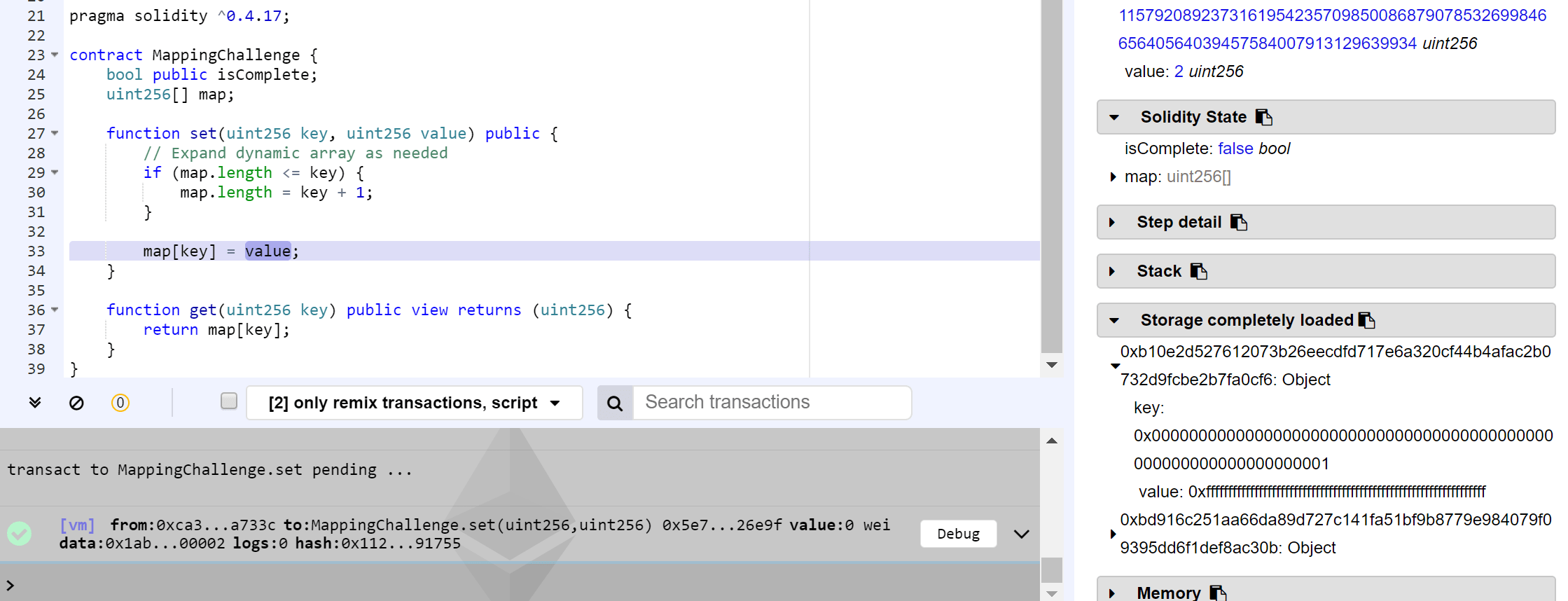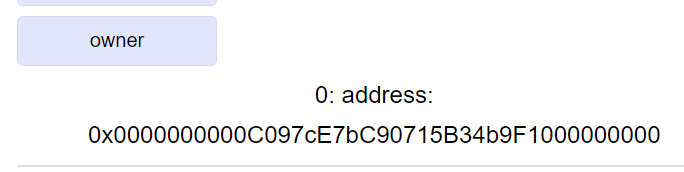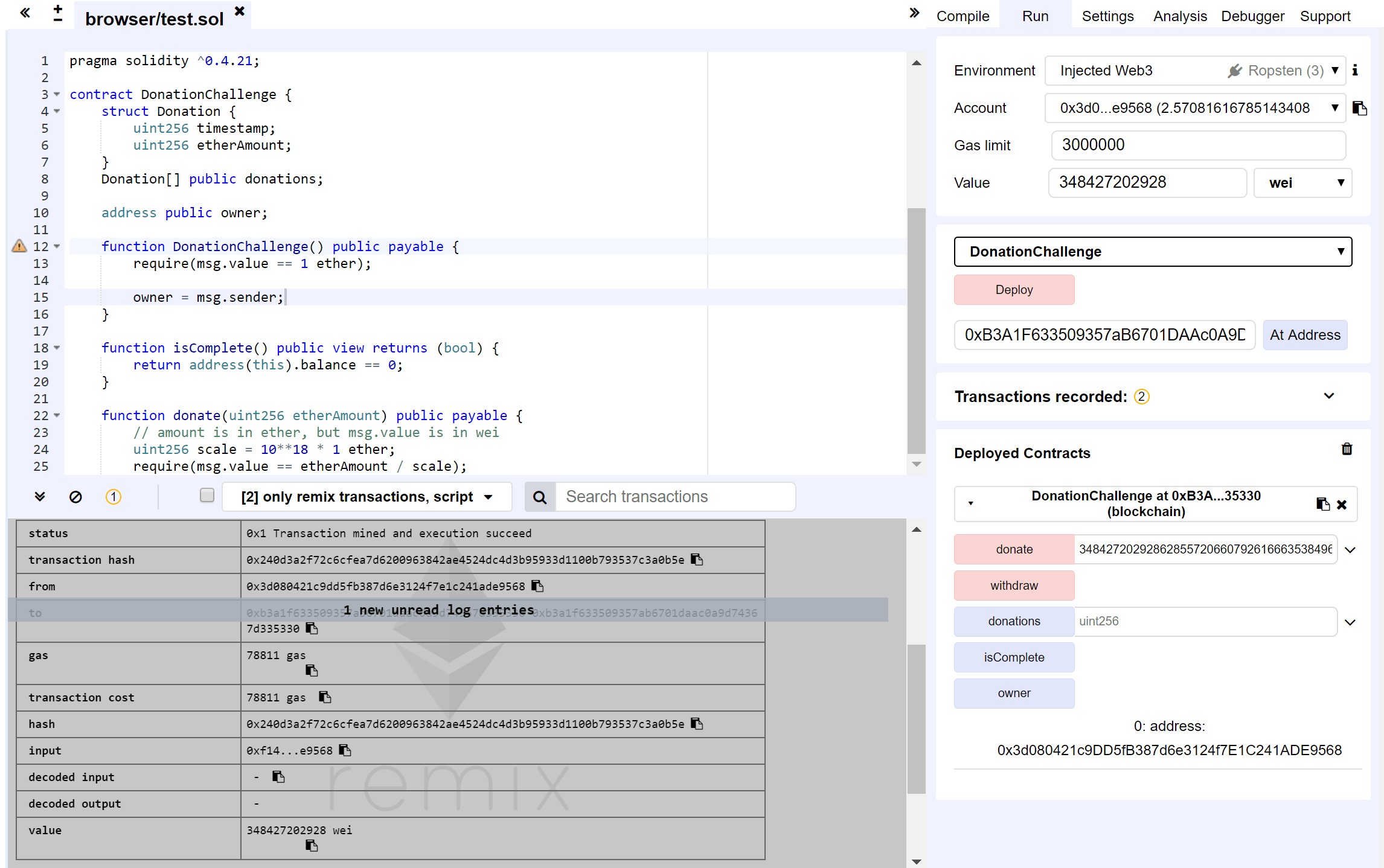# Smart Contract Exploits Part 2 (Math)

Our lead developer dishes out more hacks and solutions in this exclusive series.

### Contract Exploits Pt. 2 – Featuring Capture the Ether

Here we are with part 2 of Capture the Ether. After drafting my notes, decided that I’ll approach the Math section first, and finally wrapping up with Accounts + Miscellaneous. The Math section, like its name suggests, is focused on mostly math-based challenges, centered around overflows, manipulating Solidity storage mechanism, and also simply sloppy coding. Please do give the challenges a try if you have yet to do so, as I personally find this is where Capture the Ether starts getting really interesting.

For those who missed the first part: https://bitcoinmalaysia.com/2018/09/22/smart-contract-exploits-part-1-lotteries/

The website where these challenges could be found: https://capturetheether.com/challenges/
And the author of these challenges is the very brilliant smarx, catch him on his twitter handle @smarx.

As before, this article will require some prior knowledge with Solidity and its surrounding dev tools.

### 7. Token Sale

Source code as below.

```pragma solidity ^0.4.21;

contract TokenSaleChallenge {
uint256 constant PRICE_PER_TOKEN = 1 ether;

function TokenSaleChallenge(address _player) public payable {
require(msg.value == 1 ether);
}

function isComplete() public view returns (bool) {
}

function buy(uint256 numTokens) public payable {
require(msg.value == numTokens * PRICE_PER_TOKEN);

balanceOf[msg.sender] += numTokens;
}

function sell(uint256 numTokens) public {
require(balanceOf[msg.sender] >= numTokens);

balanceOf[msg.sender] -= numTokens;
msg.sender.transfer(numTokens * PRICE_PER_TOKEN);
}
}```

To win, we will need to drain the contract of the initial 1 ether deposit placed into the contract when it was created. There are only two functions `buy()` and `sell()` that we could access, so there must be a way for us to reach a state where we could sell more than we could buy off this contract to have it qualify for `address(this).balance < 1 ether`.

It might not be immediately apparent; It is possible to overflow the contract. The key being on the line `require(msg.value == numTokens * PRICE_PER_TOKEN)``PRICE_PER_TOKEN` being a constant of 1 ether, and all computation will be done using the figure 1,000,000,000,000,000,000. We could then overflow the check by multiplying `PRICE_PER_TOKEN` with a huge number, matching it with the equivalent modulus of msg.value, giving ourselves a huge number of `numTokens` which subsequently we could withdraw the ethers deposited into the contract to result in the value being < 1 ether.

So first we need to figure out what number we could use to reasonably overflow, allowing us to send a reasonable sum of ether across to fulfill the require check. uint256 at its maximum is 2**256 – 1, which is:

115792089237316195423570985008687907853269984665640564039457584007913129639935

We are multiplying this with 10**18, so let’s take out the last 18 digits, giving us:

115792089237316195423570985008687907853269984665640564039457

Add 1, and when we multiple this with 10**18 we will have:

115792089237316195423570985008687907853269984665640564039458000000000000000000

Which would overflow to 415992086870360064, slightly below half an ether.

Now we know the number, we just need to call `buy()` with 115792089237316195423570985008687907853269984665640564039458 as the parameter, while sending 415992086870360064 wei along with our transaction, which will overflow and give us a huge amount of tokens:

Subsequently, we can call sell with 1 to refund us the 1 ether sent to the contract, leaving 0.41…64 ethers in the contract, which will win us the challenge.

### 8. Token Whale

Source code as below.

```pragma solidity ^0.4.21;

contract TokenWhaleChallenge {

uint256 public totalSupply;

string public name = "Simple ERC20 Token";
string public symbol = "SET";
uint8 public decimals = 18;

player = _player;
totalSupply = 1000;
balanceOf[player] = 1000;
}

function isComplete() public view returns (bool) {
return balanceOf[player] >= 1000000;
}

function _transfer(address to, uint256 value) internal {
balanceOf[msg.sender] -= value;
balanceOf[to] += value;

emit Transfer(msg.sender, to, value);
}

function transfer(address to, uint256 value) public {
require(balanceOf[msg.sender] >= value);
require(balanceOf[to] + value >= balanceOf[to]);

_transfer(to, value);
}

function approve(address spender, uint256 value) public {
allowance[msg.sender][spender] = value;
emit Approval(msg.sender, spender, value);
}

require(balanceOf[from] >= value);
require(balanceOf[to] + value >= balanceOf[to]);
require(allowance[from][msg.sender] >= value);

allowance[from][msg.sender] -= value;
_transfer(to, value);
}
}```

A quick look and we can see this contract is most likely prone to an overflow vulnerability, evident by the liberal use of arithmetic operations without bound checks (e.g. SafeMath library). Though I think the main undoing of the contract is the sloppy coding, bound checks aside – `transferFrom` calls `_transfer`, which sends tokens from the msg.sender instead between the from and to addresses.

The exploit sequence will then look like below:

1. Allow a proxy account to be assigned an arbitrarily huge allowance from the player.
2. From the proxy account, execute transferFrom between the player and another account. This will overflow the balance on the proxy account, giving it a huge number of tokens.
3. From the proxy account, transfer tokens to the player to have its balance > 1,000,000.

Firing up Visual Studio to create the solution with Nethereum (likewise any other libraries are fine):

Run it and we should get:

### 9. Retirement Fund

Source code as below.

```pragma solidity ^0.4.21;

contract RetirementFundChallenge {
uint256 startBalance;
uint256 expiration = now + 10 years;

function RetirementFundChallenge(address player) public payable {
require(msg.value == 1 ether);

beneficiary = player;
startBalance = msg.value;
}

function isComplete() public view returns (bool) {
}

function withdraw() public {
require(msg.sender == owner);

if (now < expiration) {
// early withdrawal incurs a 10% penalty
} else {
}
}

function collectPenalty() public {
require(msg.sender == beneficiary);

uint256 withdrawn = startBalance - address(this).balance;

// an early withdrawal occurred
require(withdrawn > 0);

// penalty is what's left
}
}```

What happens with this contract really only focuses on `collectPenalty()`, as due to require(msg.sender == owner), where owner is the Capture the Ether factory contract, we could never call withdraw(). The focus is then on collectPenalty() to execute our exploit.

How we could exploit this contract is dependent on an EVM quirk. In essence, if we could force some ethers into the contract, making `address(this).balance > startBalance` prompting an overflow to variable withdraw, we will be able to drain every ether within this contract. The two ways of doing so is well documented in the Solidity docs:Forcing ether to a contract with a coinbase transaction or selfdestruct.

There is also a third way – as contract addresses are generated deterministically – basically rightmost 160 bits of the keccak256 result of the sender address and nonce in RLP format documented in the yellow paper as below:

So it is possible to figure out which address the Retirement Fund contract will have since we could figure out both the nonce and the address from where the contract would be deployed from; But let us just go for the easiest option here.

In a nutshell, what we need to do is to write a contract, load it up with some ethers, execute selfdestruct with the address of the contract we intend to exploit, then call `collectPenalty()`.

Subsequently, call `collectPenalty()` on the Token Whale Challenge contract, and we are done!

### 10. Mapping

Source code as below.

```pragma solidity ^0.4.21;

contract MappingChallenge {
bool public isComplete;
uint256[] map;

function set(uint256 key, uint256 value) public {
// Expand dynamic array as needed
if (map.length <= key) {
map.length = key + 1;
}

map[key] = value;
}

function get(uint256 key) public view returns (uint256) {
return map[key];
}
}```

This is a real interesting exploit and would give us a better insight onto how Solidity storage patterns work. A slight departure from the rest, this contract does not require any ether deposits, but requiring us to somehow turn `isComplete` to true. The only function allowing us to write to the contract is `set()`, so let us tackle the problem from there.

The `set()` function allows us to write to an array `map[]`, which we could specify what value and also the exact array position we’d like to write to. Now recall that the EVM deals with contract storage as a 256 bit pointer by 32 byte value slot (so 32 bytes key to 32 bytes value). Additionally, our array here is a dynamic array, which the EVM couldn’t make any assumptions of how much state storage to reserve for and therefore has a reserved slot to determine the size of the array, subsequently a keccak256 hash of the slot as the address where the value is stored. This is interesting, as it means if we could somehow expand the bound of the array to cover even the `isComplete` variable storage, we could access and overwrite the value of the variable provided we could find out which address to write to!

This in fact is a fairly well documented exploit, which won the Underhanded Solidity Coding Contest during 2017: https://github.com/Arachnid/uscc/tree/master/submissions-2017/doughoyte

Now for the exploit itself – From the exploit contract, we are allowed to write to any arbitrary location of the contract as we could specify the parameter `key`. While the USCC2017 exploit relied on an underflow to cause the array’s indices to bypass the bounds of the array, here we could instead specify the maximum of uint256 minus 2 (since the if statement will expand the array length by 1) as the input for parameter `key` to bypass the array bounds, then figuring out which address `isComplete`is by wrapping around uint256 from the hash offset of the array, which brings us to the address of storage slot 0x0.

(Note that the below example was done with compiler settings set to Solidity v0.4.17. This is important and will be explained further down below).

First, we force the array to be out of bounds using the function `set()` by entering 2**256 – 2 and a random value, say 2:Forcing array to be out-of-bounds – Note the value being the maximum of uint256.

Then, we calculate which address we need to work with to access `isComplete`. In this case, 0xb10e2d527612073b26eecdfd717e6a320cf44b4afac2b0732d9fcbe2b7fa0cf6 is the address slot where our array variable started with, so we could run the below Python script to wrap it around:

`print '0x{0:02x}'.format(2**256 - 0xb10e2d527612073b26eecdfd717e6a320cf44b4afac2b0732d9fcbe2b7fa0cf6)`

And if we access this address directly on the function `set()` with the value 1:

`isComplete` will end up returning true:

Interestingly, there is a bug fix to Solidity v0.4.22 which actually makes contract of this nature easier to hack – Which behaves to skip unneeded array storage if we point to an index larger than the existing array length (I believe it was for gas cost savings), and as we are able to figure out which address slot 0x0 is this inadvertently allowed us to push a value straight into slot 0x0. With versions older than v0.4.22, one would get an “out of gas” error when trying to jump over a huge number of array slots. The contract on CaptureTheEther was already recompiled to allow the exploit to be carried out on v0.4.22.

Let’s recompile on v0.4.22 and try this, with the same parameters 0x4ef1d2ad89edf8c4d91132028e8195cdf30bb4b5053d4f8cd260341d4805f30a and value 1:

Notice the length is automatically pushed to the desired location, and if we access isComplete:

The real takeaway of this exploit is really to ask yourself, “when a function does state changes to an array, should it be allowed to do so with an input parameter being the index of the array”?

### 11. Donation

Source code as below.

```pragma solidity ^0.4.21;

contract DonationChallenge {
struct Donation {
uint256 timestamp;
uint256 etherAmount;
}
Donation[] public donations;

function DonationChallenge() public payable {
require(msg.value == 1 ether);

owner = msg.sender;
}

function isComplete() public view returns (bool) {
}

function donate(uint256 etherAmount) public payable {
// amount is in ether, but msg.value is in wei
uint256 scale = 10**18 * 1 ether;
require(msg.value == etherAmount / scale);

Donation donation;
donation.timestamp = now;
donation.etherAmount = etherAmount;

donations.push(donation);
}

function withdraw() public {
require(msg.sender == owner);

}
}```

Ah. This one is… Horrendous. The contract by itself is so badly written it should not have passed a basic review. The `donate()` function does not work entirely and even if not noticed through a visual review, should be noticeable once the function is ran.

Basically, there are two issues:

1. The Donation struct isn’t declared properly – A pointer to the struct is attempted which results in some fairly funky behavior, namely directly accessing the contract storage slots, allowing us to overwrite other contract state variables.
2. The scale is calculated wrongly – It appears to want to scale the entered value to 1 ether, however multiplies it with 10**36, so we can send a msg.value which is effectively `etherAmount / 10**36`, a fraction of what I assume the contract would really like to receive.

Let’s test to see what behaviour can be invoked. Let’s try sending in 1 wei, with `etherAmount` = 10**36.

THE HORROR. The entire Owner variable got overwritten!Hmmmmm. c097ce7bc90715b34b9f1000000000 is not the original owner.

If we look closer at the code on Remix, Remix was already throwing a warning (as below). Basically, declaring donation this way creates a pointer direct to contract storage, instead of a temporary memory store which the code later pushes to the donations array.

In other words, writing to `donation.timestamp` and `donation.etherAmount` in reality writes to storage pointer 0 and pointer 1, where storage pointer 1 reflects the variable `Owner`, giving us the ability to manipulate this variable.

And how do we use this to our advantage? The hex c097ce7bc90715b34b9f1000000000 refers to the etherAmount we have entered, being 10**36. In this case, we can edit the Owner variable by figuring out what is the uint256 equivalent of our address using a hex to decimal converter, pass it as a parameter for `etherAmount`, while sending in msg.value equivalent to `etherAmount / 10**36`, effectively giving our address access to withdraw all funds from the contract.Convert your address from hex to decimal, using it as a parameter.

All these could be circumvented by declaring Donation memory `donation` (and also having `scale` properly reflect 10**18 if we want to reflect the donated amount correctly).

### 12. Fifty Years

Source code as below.

```pragma solidity ^0.4.21;

contract FiftyYearsChallenge {
struct Contribution {
uint256 amount;
uint256 unlockTimestamp;
}
Contribution[] queue;

function FiftyYearsChallenge(address player) public payable {
require(msg.value == 1 ether);

owner = player;
queue.push(Contribution(msg.value, now + 50 years));
}

function isComplete() public view returns (bool) {
}

function upsert(uint256 index, uint256 timestamp) public payable {
require(msg.sender == owner);

if (index >= head && index < queue.length) {
// Update existing contribution amount without updating timestamp.
Contribution storage contribution = queue[index];
contribution.amount += msg.value;
} else {
// Append a new contribution. Require that each contribution unlock
// at least 1 day after the previous one.
require(timestamp >= queue[queue.length - 1].unlockTimestamp + 1 days);

contribution.amount = msg.value;
contribution.unlockTimestamp = timestamp;
queue.push(contribution);
}
}

function withdraw(uint256 index) public {
require(msg.sender == owner);
require(now >= queue[index].unlockTimestamp);

// Withdraw this and any earlier contributions.
uint256 total = 0;
for (uint256 i = head; i <= index; i++) {
total += queue[i].amount;

// Reclaim storage.
delete queue[i];
}

// Move the head of the queue forward so we don't have to loop over

msg.sender.transfer(total);
}
}```

The highest scored of the entire CaptureTheEther challenge at 2000 points. The description below on how to exploit this contract will be rather long, so please bear with me.

In essence the exploits themselves aren’t exactly different than what we have already experienced so far, however this contract requires the correct sequence of executing a few exploits before we could drain it dry.

Starting with a few observations:

1. The “else” statement on the `upsert()` function does not properly declare the contribution variable, instead relies on the earlier declaration in the “if” statement, which creates a pointer to the struct. This means… Again, we have the opportunity to exploit the contract storage slots 0 and 1 directly.
2. The line `queue[queue.length - 1].unlockTimestamp + 1` days could potentially be overflowed to our advantage.
3. The code `queue.push` on the “else” statement is supposed to push a copy (memory) of the contribution struct to the existing array. Since `contribution.amount` and `contribution.unlockTimeStamp` results in us accessing storage slots directly… What will we end up pushing to array `queue` here?

Let’s confirm our observations.

1. Entering a value pair where the index isn’t 0 and `timestamp = queue.timestamp + 86400` with a msg.value of 0, overwrites slot 0x0 with 0 and 0x1 with timestamp + 86400. These map to `queue`‘s length and variable `head` respectively.

This means if we would like to retain `queue`‘s array length, we need to also increment the msg.value when we call `upsert()`.

2. This one is straightforward. Enter something with a timestamp of 2**256 – 86400, and the next round we could enter 0 as our timestamp. Remember that we need to send in an incrementing number of wei (msg.value) each time we do this with `upsert()`, to allow us to appropriately retain the length of the array each time we push a new element.
3. Without specifying a msg.value, if we execute an `upsert()` with index 1 and timestamp + 86400, we can see that the value 1 for amount and timestamp + 86400 is being pushed to the array. Continuing this with index 2 and timestamp + 172800, we can see 1 and timestamp + 172800 being pushed to the array. On the other hand, if we repeat the same thing while specifying an incrementing msg.value to increase the array length as we push new elements, we see each element’s amount increasing in line with the length of the array (or more accurately, based off msg.value + 1, since it increments the array length by msg.value then push the array with the latest element).

Knowing all these, let us see how we could piece together an exploit for this contract. Examining the code for the `withdraw()` function, we can see we are allowed to pass in the index we would like to withdraw till, provided that the timestamp of that index has expired (passing current time). The variable `head` is used here to prevent us from looping through indices we have already withdrawn from. Therefore, at the very minimal to exploit the contract we need to fulfill the conditions a) have the timestamp of the index we’d like to withdraw from expired b) Have head set to 0 so we could withdraw from the very initial contribution. To arrive to this state, we could execute a sequence as such:

1. Call `upsert()` with index = 1 (amount becomes 2), timestamp = 2**256 – 86400, with msg.value = 1 wei.
Result is the element gets appended to the queue array (queue.length = 2), and contract holds a total of 1 ether and 1 wei. Variable `header` will be 2**256 – 86400.
2. Call `upsert()` with index = 2 (amount becomes 3), timestamp = 0, with msg.value = 2 wei.
Result is the element gets appended to the queue array (queue.length = 3), and the contract holds a total of 1 ether and 3 wei. Variable `header` will be 0.
Note that at this point, we can’t withdraw from the contract yet as the amount total (10**18 + 2 + 3) is more than the actual value (1 ether 3 wei) held by the contract. So we need to get more ethers into the contract, and withdraw from an earlier index, and attempt to drain the remainder as a separate process.
3. Call `upsert()` with index = 3 (amount becomes 4), timestamp = 86400, with msg.value = 3 wei.
Result is the element gets appended to the queue array (queue.length = 4), and the contract holds a total of 1 ether and 6 wei. Variable `header` will be 86400.
4. Call `upsert()` with index = 4 (amount becomes 5), timestamp = 2**256 – 86400, with msg.value = 4 wei.
Result is the element gets appended to the queue array (queue.length = 5), and the contract holds a total of 1 ether and 10 wei. Variable `header` will be 2**256 – 86400.
5. Call `upsert()` with index = 5 (amount becomes 6), timestamp = 0, with msg.value = 5 wei.
Result is the element gets appended to the queue (array.length = 6), and the contract holds a total of 1 ether and 15 wei. Variable `header` will be 0.
6. Now, we can call `withdraw()` on index 3. This allows us to withdraw 1 ether + 2 wei + 3 wei + 4 wei, leaving the contract with 6 wei to be drained.

Now we have successfully drained the contract. Note that `returnTotal` is not present by default – Was added for me to quickly check the contract balance while testing.

How do we drain the remainder 6 wei? Recall that we could execute an `upsert()` without sending in msg.value, which will result in the `contribution.amount` being 1. What we need to figure out is how do we reach a state where `contribution.timestamp` is 0 when `contribution.amount is 1`. This can be done simply by alternating between timestamp 2**256 – 86400 then 0, which allows us to arrive to `contribution.amount = 1` and `contribution.timestamp = 0`. Subsequently, withdraw on index 0. Repeat these actions 6 times and we will be able to drain this contract entirely!

This entire sequence may seem a bit abstract and hard to follow, so I do have the below Gist link which shows how I executed the exploit, using Nethereum, in VB.NET: https://gist.github.com/Enigmatic331/1af7f92d221bd831fc81f50ac8cd72ea

### Conclusion

This wraps up the second part of this multi-part series. Keeping value on a smart contract can be risky, and the more value it keeps, the more eyes which vet through the contract the better. A few key takeaways:

1. Always have an extra pair of eyes to review through your code; If there is no one else who could help you then be as thorough as possible – review, unit test, review,try to break your own code, rinse and repeat.
2. If you are developing production code meant to store huge amount of value, the best option is to engage smart contract auditors like ChainSecurity and have your contract professionally audited.
3. Use SafeMath for arithmetic operations. Period.
4. Do not rely on user input for array index allocation. Code so this could be handled by the smart contract internally.

The last part to these series will come in a couple of weeks, where a challenge or two is slightly outside the scope of simply hacking a smart contract. Will be interesting. And… If you have not yet, I would still encourage you to try your hand on the rest of the challenges in the meantime – All the best!

This site uses Akismet to reduce spam. Learn how your comment data is processed.